# Tecplot Tip: How to insert CAD drawing into 3D flow field data

This Tip describes imposing a 3D wing model on 3D flow field in Tecplot 360. The 3D flow field data are obtained using Volumetric Three-Component Velocimetry (V3V) where the three components of the velocity vectors are measured in a 3D volume of 140X140X100 mm3. Figure 1 shows slices in x-z and y-z planes of the streamwise mean velocity over a periodically cambered plate at an angle of attack of 8 degrees at Reynolds number of 28,000. The data is shown in Tecplot 360.

This Tecplot Tip was contributed by: Dr. Redha Wahidi and Mr. Zheng Zhang (PhD candidate), Dept. of Aerospace Engineering, The University of Alabama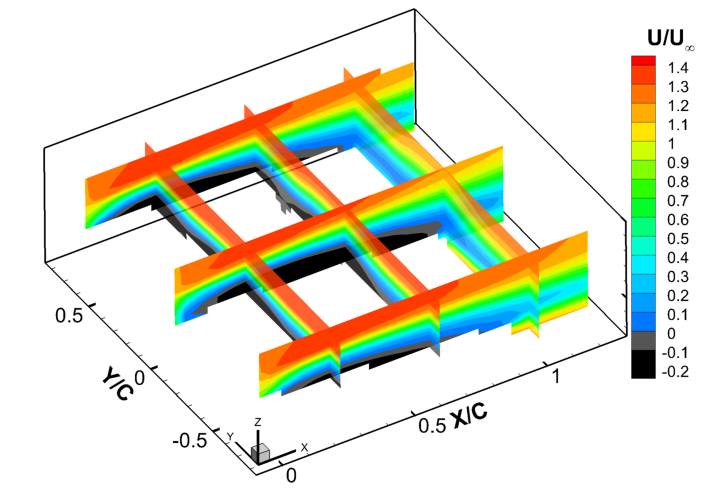Figure 1. Slices shown in x-z and y-z planes of the streamwise mean velocity over a periodically cambered plate at an angle of attack of 8 degrees at Reynolds number of 28,000.

## Follow this procedure to include the test article geometry with the 3D flow field in Tecplot:

1. Create a 3D drawing of the wing model using SolidWorks and save it in STL format. To facilitate easier alignment between the 3D drawing and 3D flow field data in Tecplot, we recommend matching the dimensions and coordinate system in the 3D drawing to the 3D flow field data. This means that the distances in the x, y and z directions should match. Also, the origin and orientation of coordinate system in the 3D drawing and flow field data should match. In the example shown (Figure 2), the origin is located at the midspan leading-edge location. Before saving the file in STL format, click the “option” dialog in SolidWorks and examine the setting and ensure that the “Do not translate STL output to positive space” option is checked (Figure 3).Figure 2. The origin is located at the midspan leading-edge location.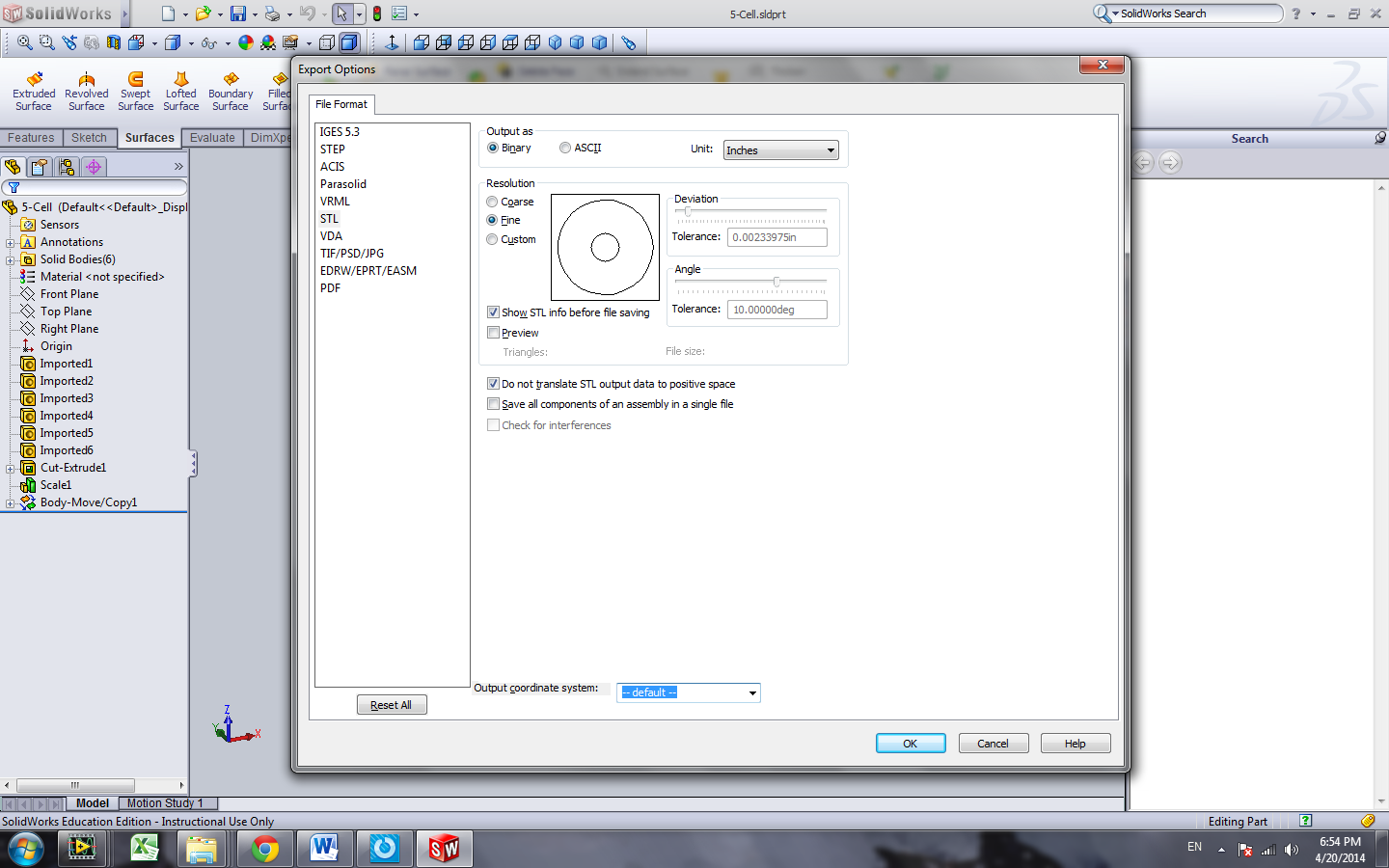Figure 3. Make sure that the “Do not translate STL output to positive space” option is checked.

2. Open a Tecplot file that contains the flowfield data.
3. Go to “Create New Frame” in the “Frame” menu.
4. Draw a “box” anywhere in the layout.
6. Select “3D System STL”
7. Browse to find the CAD drawing.
8. Select “3D Cartesian” from the “Initial Plot Type” drop down menu. Figure 4 shows the CAD drawing with the flow field data.
9.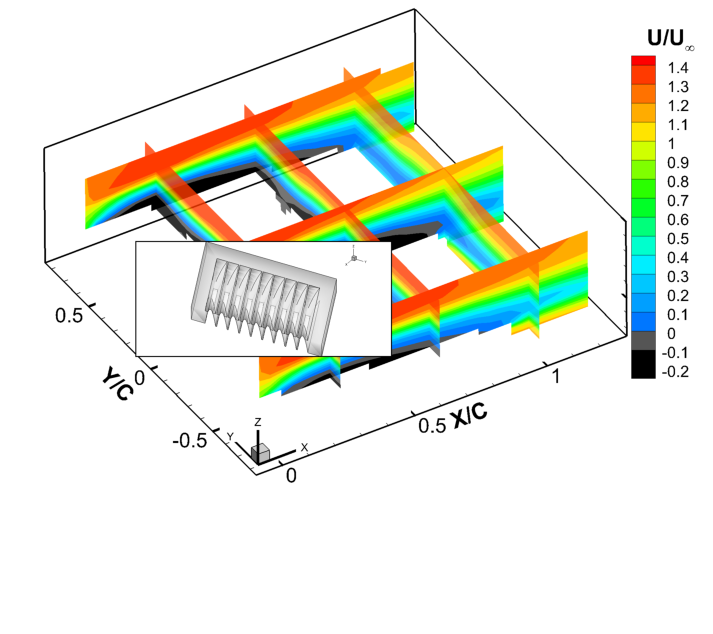Figure 4. CAD drawing with the flow field data.

11. Check “Frame Size and Position” and “3D Plot View.”
12. Select “Order Frame” from the “Frame” menu, and select “Activate” Frame 002.
14. Check “Frame Size and Position” and “3D Plot View.”
15. Pop Frame 001 and activate it.
16. Select “Edit Active Frame” in the “Frame” menu and uncheck “Show Background.”
17. Remove the axes from the current active frame, i.e., Frame 001.
18. Activate Frame 002 and show the axes on this frame.
19. Adjust the size and position as desired. Figures 5 and 6 show the flow field data with the wing model. Figure 5 shows the streamwise mean velocity with the wing model. Figure 6 shows iso-surfaces of the normal component of the vorticity vector with the wing model.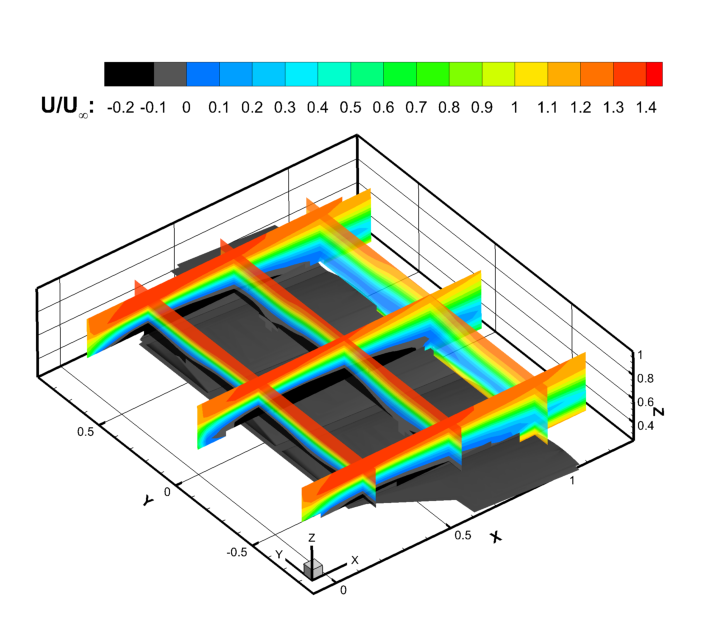Figure 5. Streamwise mean velocity with the wing model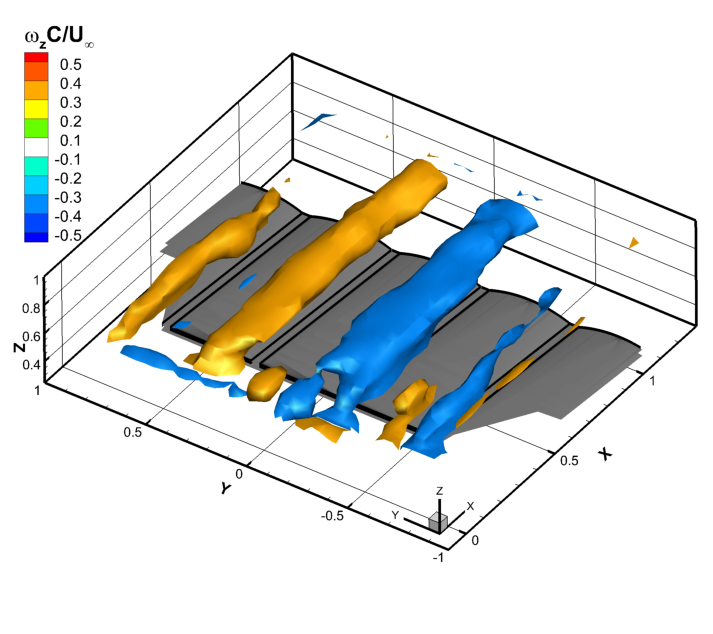Figure 6. Iso-surfaces of the normal component of the vorticity vector with the wing model.

Do you have a Tecplot Tip to share? We would love to hear from you. Email your tip to marketing@tecplot.com.